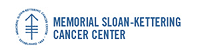Memorial Sloan-Kettering Cancer Center, Dept. of Epidemiology & Biostatistics Working Paper Series

Abstract

Receiver operating characteristic (ROC) curves are useful in evaluating the ability of a continuous marker in discriminating between the two states of a binary outcome such as diseased/not diseased. The most popular parametric model for an ROC curve is the binormal model which assumes that the marker is normally distributed conditional on the outcome. Here we present an alternative to the binormal model based on the Lehmann family, also known as the proportional hazards specification. The resulting ROC curve and its functionals (such as the area under the curve) have simple analytic forms. We derive closed-form expressions for the asymptotic variances of the estimators for various quantities of interest. This family easily accommodates comparison of multiple markers, covariate adjustments and clustered data through a regression formulation. Evaluation of the underlying assumptions, model fitting and model selection can all be performed using any off the shelf proportional hazards statistical software package.

Biostatistics

COinS